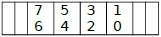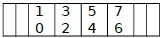# How do I convert between big-endian and little-endian values in C++?

Here we will see how to convert Little endian value to Big endian or big endian value to little endian in C++. Before going to the actual discussion, we will see what is the big endian and the little endian?

In different architectures, the multi-byte data can be stored in two different ways. Sometimes the higher order bytes are stored first, in that case these are known as big endian, and sometimes the lower order bytes are stored first, then it is called little endian.

For example, if the number is 0x9876543210, then the big endian will be −The little endian will be like this −In this section we will see how to convert little endian to big endian and vice versa. To do this we have to interchange the 1st and 4th bytes, and 2nd and 3rd bytes. We can interchange them using logical expressions. Make Copy of the number four times, then for the first copy, shift the 1st byte 24 times to the right, for the second copy, mask it with 00FF0000, then swap 8 bits to the right, for the 3rd copy mask it with 0000FF00, then shift left 8 bits, and for the last copy swap the elements to the left 24 times. Then Logically OR these four copies to get the reversed result.

## Example

#include <iostream>
#define SWAP_INT32(x) (((x) >> 24) | (((x) & 0x00FF0000) >> 8) | (((x) & 0x0000FF00) << 8) | ((x) << 24)) using namespace std;
void memory_represent(char *value, int n) {
int i;
for (i = 0; i < n; i++)
printf(" %.2x", value[i]);
}
int main() {
unsigned int x = 0x9876543210;
int y;
cout << "The little endian value is: ";
memory_represent((char*)&x, sizeof(x));
cout << endl;
y = SWAP_INT32(x);
cout << "The Big endian value after conversion is: ";
memory_represent((char*)&y, sizeof(y));
cout << endl;
}

## Output

The little endian value is: 10 32 54 76
The Big endian value after conversion is: 76 54 32 10

Updated on: 30-Jul-2019

5K+ Views